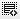## How to calculate the flat rate paid on a loan0
Calculate the flat rate paid on a loan of Rs. 20,000 that is repaid over 5 years. Payments are made monthly. Each payment is Rs.500.

10%

12%

9%

11%

THIS WAS NOT DISCUSSED IN VIDEO KINDLY LET ME KNOW HOW TO SOLVE I AM FINDING IT DIFICULT TO UNDERSTAND FLAT RATE0

Hi, hope you received our email - when would be a good time for you to have a call with our faculty to clear all your doubts.0

Hi !
Flat rate is simply the simple interest charged over the period of loan. In the above question, the monthly payment is Rs. 500 and period of loan is 5 years or 60 months, so the total repayment will be = Rs.500*60 = Rs. 30,000. And hence, total interest paid will be = 30,000-20,000= 10,000. This is equal to 50% of 20,000 or the loan amount and for 5 years. Therefore to get the annual flat rate we simply divide by 5 i.e. =50%/5 = 10%. Which is the answer.

Click on thisicon to add code snippet.